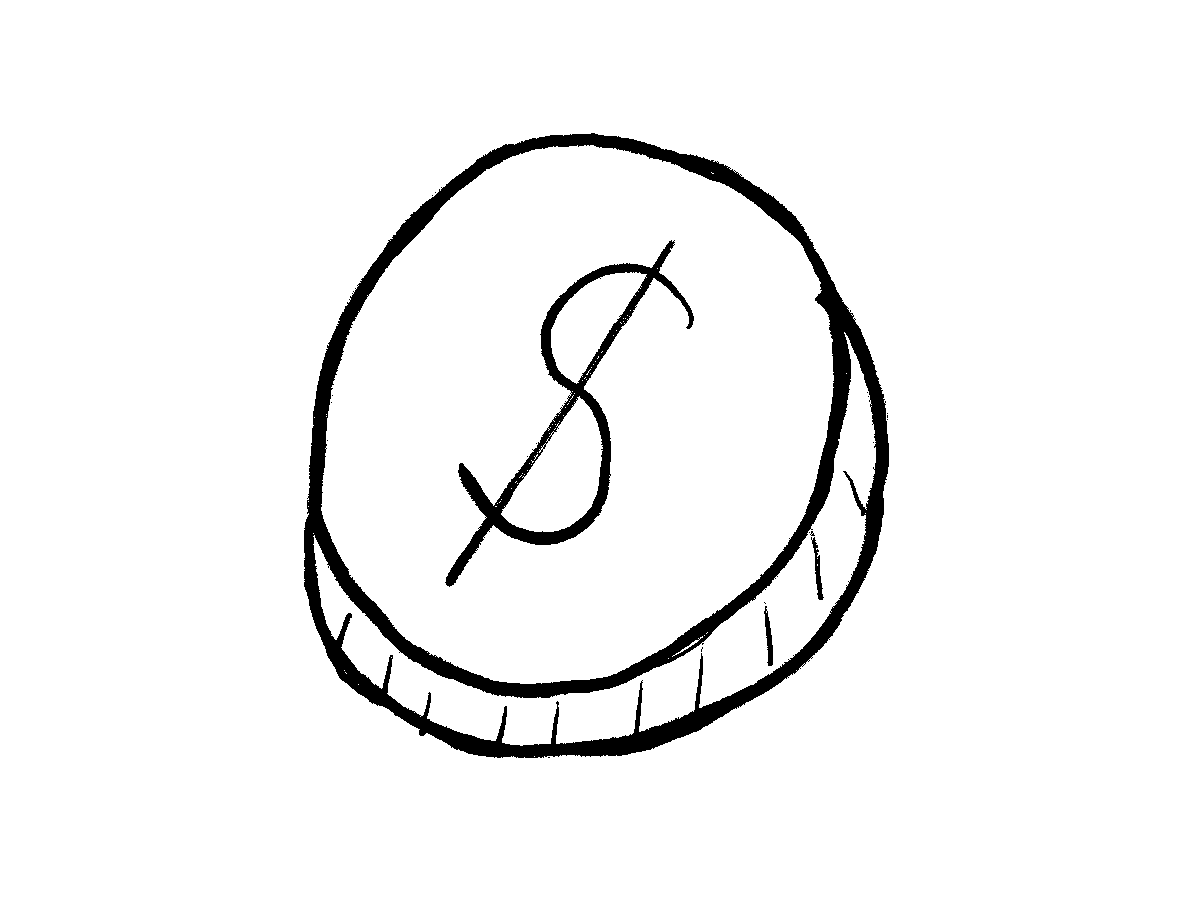# You have three coins in your pocket…

Solving a puzzle from 538’s Riddler Express.

Miha Gazvoda https://mihagazvoda.com
2021-03-07

This weekend I’ve been solving the March 5 538’s Riddler Express:

You have three coins in your pocket, each of which can be a penny, nickel, dime or quarter with equal probability. You might have three different coins, three of the same coin or two coins that are the same and one that is different.

Each of these coins can buy you a string whose length in centimeters equals the value of the coin in cents, i.e., the penny buys 1 cm of string, the nickel buys 5 cm of string, etc. After purchasing your three lengths of string, what is the probability that they can be the side lengths of a triangle?

Although the analytical solution is simple, I chose the simulation. More fun!# Solution

The possible coin values, i.e. lengths of strings we can buy with the coins:1

``````coin_sample_space <- c(1L, 5L, 10L, 25L)
``````

With the `sample` function, we sample with replacement three coins that will be in our pocket.

``````sample(coin_sample_space, size = 3, replace = TRUE)
``````
`` 10  5  5``

With these three coins, we buy strings of appropriate lengths. To determine whether the strings can shape a triangle, the sum of the shorter two has to be longer than the longest one.

``````is_triangle <- function(coins) {
sum(coins) > 2 * max(coins)
}
``````

Note that `sum(coins)` already contains maximum length - that’s why we are comparing it to two times the max length.

We repeat the same process zillion, okay `n`, times:

1. Sample three coins with replacement from `coin_sample_space`.
2. Determine whether we can shape a triangle with the purchased strings.
``````n <- 1e5

are_triangles <- replicate(
n,
is_triangle(sample(coin_sample_space, size = 3, replace = TRUE))
)

mean(are_triangles)
``````
`` 0.34061``

In around 34% of cases, we can shape the triangle. In the rest, we will have to live without it.

1. `L` indicates integer.↩︎